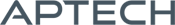# FANPAC MT

FANPAC MT

Availability: Out of stock

FANPAC MT is designed for estimating parameters of univariate and multivariate Generalized Autoregressive Conditionally Heteroskedastic (GARCH) models.
Overview

## FANPAC MT

The Financial Analysis Application MT (FANPAC MT) provides econometric tools commonly implemented for estimation and analysis of financial data. FANPAC MT allows users to tailor each session to their specific modeling needs and is designed for estimating parameters of univariate and multivariate Generalized Autoregressive Conditionally Heteroskedastic (GARCH) models.

Platform: Windows, Mac, and Linux

Requirements: GAUSS/GAUSS Engine/GAUSS Light v10 or higher

Features

## Supported models:

• BEKK GARCH model
• Diagonal VEC multivariate models:
• GARCH model
• Fractionally integrated GARCH model
• GJR GARCH model
• Multivariate constant conditional correlation models:
• GARCH model
• Exponential GARCH model
• Fractionally integrated GARCH model
• GJR GARCH model
• Multivariate dynamic conditional correlation models:
• GARCH model
• Exponential GARCH model
• Fractionally integrated GARCH model
• GJR GARCH model
• Multivariate factor GARCH model
• Generalized orthogonal GARCH model
• Univariate time series models:
• GARCH model
• OLS
• ARIMA

## Modeling Flexibility Provided with User-Specified Modeling Features:

• GARCH, ARCH, autoregressive, and moving average orders
• Flexible enforcement of stationarity and nonnegative conditional variance requirements
• Pre-programmed, user controlled Boxcox data transformations
• Error density functions (Normal, Student’s t, or skew t-distribution)

## GAUSS FANPAC MT Output Includes:

• Estimates of model parameters
• Moment matrix of parameter estimates
• Confidence limits
• Time series and conditional variance matrices forecasts

### FANPAC MT Tools Facilitate Goodness-of-Fit analysis:

• Reported Akaike and Bayesian information criterion
• Computed model residuals
• Computed roots of characteristic equations
• GARCH time series data simulation
• Andrews simulation method statistical inference
• Time series ACF and PACF computation and plotting
• Data and diagnostic plots including:
• Standardized residuals
• Conditional correlations, standard deviations, and variance
• Quantile-quantile plots
• Residual diagnostics including skew, kurtosis, and Ljung-Box statistics

Examples

### Examples

Product Inquiry

4 + 6 = enter the result ex:(3 + 2 = 5)

﻿